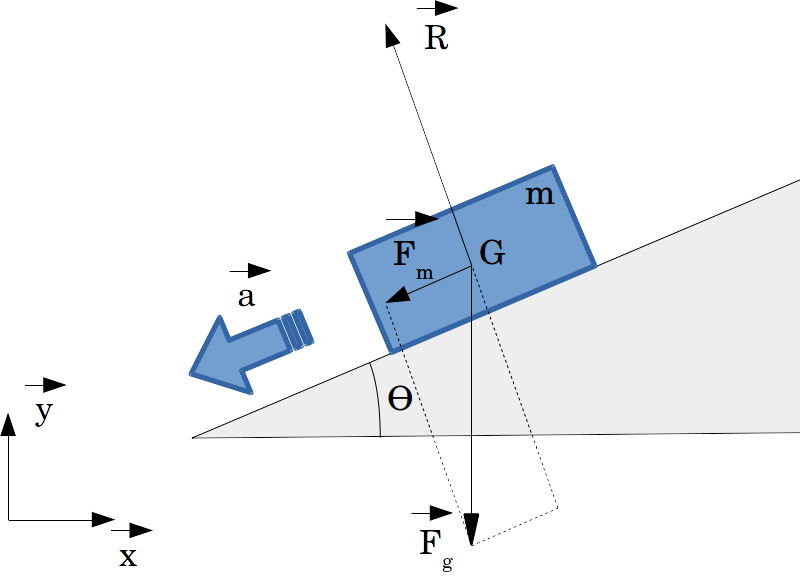Do me a favor, take a few seconds to have a look at my last project.

Thank you, Lulu

Fundamental principle of dynamics

Introduction

The second Newton's law of motion (fundamental principle of dynamics) say that the sum of the forces $$\vec{F}$$ on an object is equal to the mass $$m$$ of this object multiplied by the acceleration $$\vec{a}$$ of this object:

$$\sum{\vec{F}}=m.\vec{a}$$

This post illustrates the fundamental principle of dynamic on a very basic and understandable example. In the following, we will assume a mobile body moving on an inclined plane:The aim is to calculate the body's acceleration. We will assume the following hypotheses:

• The frictions are neglected.
• The force acting on the body is the gravity on Earth's surface $$\vec{g}$$.
• $$G$$ is the center of gravity of the body.
• $$m$$ is the body's mass.
• $$\theta$$ is the angle of the inclined plane.

Simplest approach

This first approach is the simplest, it assumes that the body can only move in the direction of the inclined plane. According to this, all the forces can be projected along the plane direction. Applying the fundamental principle of dynamics gives:

$$m.\vec{a} = \sum{\vec{F}} = \vec{R} + \vec{F_g} = \vec{F_m}$$

The forces acting on the body are the gravity force $$\vec{F_g}$$ and the ground reaction force $$\vec{R}$$. As $$\vec{R}$$ is perpendicular to the motion, its projection along the motion direction is null. The projection of $$\vec{F}$$ along the motion direction is given by:

$$| \vec{F_{m}} | = |\vec{F_g}| . sin(\Theta)$$

We can now deduce that :

$$m.|\vec{a} | = |\vec{F_g}| . sin(\Theta) = m.g.sin(\Theta)$$

Note that the previous equatio n can be divided by $$m$$. This shows that the motion is independent from the mass. Most people think that the heavier the body is, the fastest it will fall. But in fact, the falling speed is only dependent of frictions and gravity. The acceleration is thus given by:

$$| \vec{a} | = g.sin(\Theta)$$

If the plane is horizontal, $$\Theta=0$$, the body's acceleration is null, the body doesn't move. If the plane is vertical, $$\Theta=\pi/2$$, the body's acceleration is equal to g, the body is in free fall.

General approach

In the previous section, we made an assumption on the acceleration direction. In the following, we will consider that the acceleration direction is unknown. The forces acting on the body are the gravity force $$\vec{F_g}$$ and the ground reaction force $$\vec{R}$$. We will project each force vector along the $$\vec{x}$$ and $$\vec{y}$$ axes. Projection of the gravity force:

$$\vec{F_g} = \left( \begin{array}{r c l} \vec{F_x} \ \vec{F_y} \end{array} \right) = \left( \begin{array}{r c l} 0 \ -m.g \end{array} \right)$$

Projection of the ground reaction force:

$$| \vec{R} | = m.g.cos(\Theta)$$

$$\vec{R} = \left( \begin{array}{r c l} \vec{R_x} \ \vec{R_y} \end{array} \right) = \left( \begin{array}{r c l} -m.g.cos(\Theta).sin(\Theta) \ m.g.cos(\Theta).cos(\Theta) \end{array} \right)$$

As previously, the fundamental principle of dynamics gives:

$$m.\vec{a} = \sum{\vec{F}} = \vec{R} + \vec{F_g} = \vec{F_m}$$

$$m.\vec{a} = \left( \begin{array}{r c l} \vec{F_x}+\vec{R_x} \ \vec{F_y}+\vec{R_y} \end{array} \right) = \left( \begin{array}{r c l} -m.g.cos(\Theta).sin(\Theta) \ -m.g+m.g.cos(\Theta).cos(\Theta) \end{array} \right)$$

$$\vec{a} = g.\left( \begin{array}{r c l} -cos(\Theta).sin(\Theta) \ cos(\Theta)^2-1 \end{array} \right) = g.\left( \begin{array}{r c l} -sin(\Theta).cos(\Theta) \ -sin(\Theta).sin(\Theta) \end{array} \right)$$

This result confirms that the acceleration is given by:

$$| \vec{a} | = g.sin(\Theta)$$